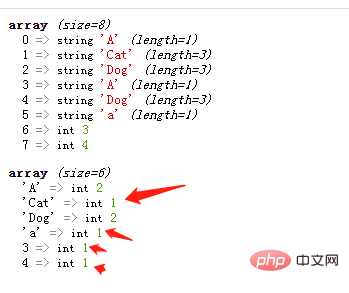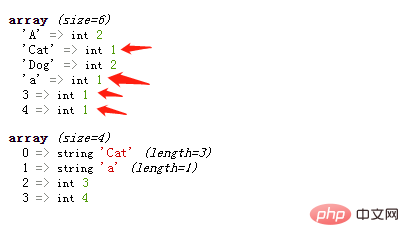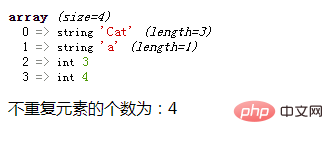# php怎么获得数组中不重复元素的个数php入门到就业线上直播课：进入学习

array_count_values() 函数可以统计数组中所有值出现的次数；如果次数为1，则该元素就不重复。

array_count_values() 函数会返回一个关联数组，其元素的键名是原数组的值，键值是该值在原数组中出现的次数。

```<?php
\$arr=array("A","Cat","Dog","A","Dog","a",3,4);
var_dump(\$arr);
\$count=array_count_values(\$arr);
var_dump(\$count);
?>``````\$result=[];
foreach(\$count as \$k=>\$v){
if(\$v==1){
\$result[]=\$k;
}
}
var_dump(\$result);``````\$len=count(\$result);
echo "不重复元素的个数为：".\$len;```1/1